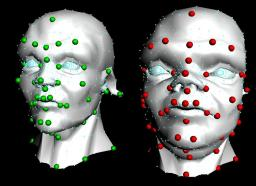## Recommended Posts

Hi all,

Been away for so long, this is my first post in some years I believe.

Anyway I was at a Houdini event the other day and they were talking through all the great new features in Houdini 13 and beyond and I noticed on one of the slides that numpy is now included in Houdini since 12.5 or even earlier. It's been so long since I delved into Houdini that this massively useful fact had totally passed me by.

The first thing that lept into my head when I saw this was my old HDK sop RBF morpher which is a totally awesome deformer that has gotten me out of so many tricky deformation scenarios over the years. Finally I realised I could re-write this in a Python sop and not have the issue of people needing to compile it to use it.

A few days later and after a couple of hours work here is the result. No error checking yet just the bare bones of the functionality.

Of course it's no where near the speed of the HDK one, but hey at least compilers aren't an issue with this one.This is the source code and I've attached a example hip file and otl, non-commercial versions.

`# This code is called when instances of this SOP cook.import numpy as npimport mathnode = hou.pwd()geo = node.geometry()inputs = node.inputs()kernel = node.parm('kernel').eval()power = node.parm('power').eval()scale = node.parm('scale').eval()def linear(r):return rdef smooth(r):return r*r*math.log1p(r*r)def cube(r):return r*r*rdef thinPlate(r):return r*r*math.log1p(r)def sqrt(r):return math.sqrt(r)def pow(r):return math.pow(r,power)kernels = {0: linear, 1: smooth, 2: cube, 3: thinPlate, 4: sqrt, 5: pow}def rbfU(r):return kernels[kernel](r)if len(inputs) > 2:featureGeo = inputs.geometry()targetGeo = inputs.geometry()numFeaturePoints = len(featureGeo.iterPoints())matrixQ = np.zeros((numFeaturePoints,4))#setup all the matrices with the feature point positionsi = 0;for p in featureGeo.points():	 matrixQ[i,0] = p.position()	 matrixQ[i,1] = p.position()	 matrixQ[i,2] = p.position()	 matrixQ[i,3] = 1	 i += 1#print matrixQmatrixQtranspose = matrixQ.transpose()#print matrixQtransposematrixK = np.zeros((numFeaturePoints, numFeaturePoints))#scale = 1for row in range(numFeaturePoints):	 for col in range(numFeaturePoints):		 ppt = featureGeo.iterPoints()[row]		 p1 = ppt.position()		 ppt = featureGeo.iterPoints()[col]		 p2 = ppt.position()		 p = p1-p2		 matrixK[row,col] = rbfU(p.length()/scale)#print matrixK#setup the final set of linear equations in one massive matrixmatrixA = np.zeros((numFeaturePoints+4, numFeaturePoints+4))for row in range(numFeaturePoints):	 for col in range(numFeaturePoints):		 matrixA[row,col] = matrixK[row,col]for row in range(numFeaturePoints):	 for col in range(numFeaturePoints, numFeaturePoints+4):		 matrixA[row,col] = matrixQ[row,col-numFeaturePoints]for row in range(numFeaturePoints, numFeaturePoints+4):	 for col in range(numFeaturePoints):		 matrixA[row,col] = matrixQtranspose[row-numFeaturePoints,col]#print matrixA#setup the solutions to all the linear equations, i.e. the target feature positionstargetX = np.zeros((numFeaturePoints+4))targetY = np.zeros((numFeaturePoints+4))targetZ = np.zeros((numFeaturePoints+4))i = 0;for p in targetGeo.points():	 targetX[i] = p.position();	 targetY[i] = p.position();	 targetZ[i] = p.position();	 i += 1#solve the linear equations to find the weights that map the features to the targetsweightsX = np.linalg.solve(matrixA, targetX)weightsY = np.linalg.solve(matrixA, targetY)weightsZ = np.linalg.solve(matrixA, targetZ)#print weightsX#apply the weights to the actual points on the input geometry to get the final resulting positions relative to the target feature pointsNfPts = numFeaturePointsfor opt in geo.points():	 outX = weightsX[NfPts]*opt.position() + weightsX[NfPts+1]*opt.position() + weightsX[NfPts+2]*opt.position() + weightsX[NfPts+3];	 outY = weightsY[NfPts]*opt.position() + weightsY[NfPts+1]*opt.position() + weightsY[NfPts+2]*opt.position() + weightsY[NfPts+3];	 outZ = weightsZ[NfPts]*opt.position() + weightsZ[NfPts+1]*opt.position() + weightsZ[NfPts+2]*opt.position() + weightsZ[NfPts+3];	 p1 = opt.position()	 i = 0	 for p2 in featureGeo.points():		 p = p1-p2.position();		 rF = rbfU(p.length()/scale);		 outX += weightsX[i]*rF		 outY += weightsY[i]*rF		 outZ += weightsZ[i]*rF		 i+=1	 opt.setPosition((outX,outY,outZ))[/CODE]`

ExampleRBF.hipnc

RBFmorpher.otl

Edited by sibarrick
•5

##### Share on other sites

As a comparison too, here is the same thing with a switch to compare to the CHOP method. Clearly way faster and if you don't need to get into the code to do anything different then probably the better solution in most cases for basic deformation....

RBFmorpher.otl

ExampleRBF.hipnc

Edited by sibarrick
•4

## Create an account

Register a new account